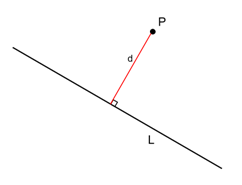Q

# Solve! - Three Dimensional Geometry - JEE Main-6

Perpendicular distance of (1,1,1) from the line   is

• Option 1)

• Option 2)

• Option 3)

• Option 4)

132 Views

As we have learned

Perpendicular distance of a point from a line, foot of perpendicular and image (Cartesian form ) - - whereinL be the foot of perpendicular drawn from on the line

Co-ordinates of L will be

DR's of PL will be

PL is perpendicular to line,

Find and put in coordinates of L Distance between P and L is perpendicular distance Image Q can be obtained by applying mid point formula in P and Q and equating it to L obtained.Let foot of perpendicular be L

So, L will be

DR of the PL will be

PL is perpendicular to given line so

L(3,0,2)

Option C

Option 1)

Option 2)

Option 3)

Option 4)

Exams
Articles
Questions